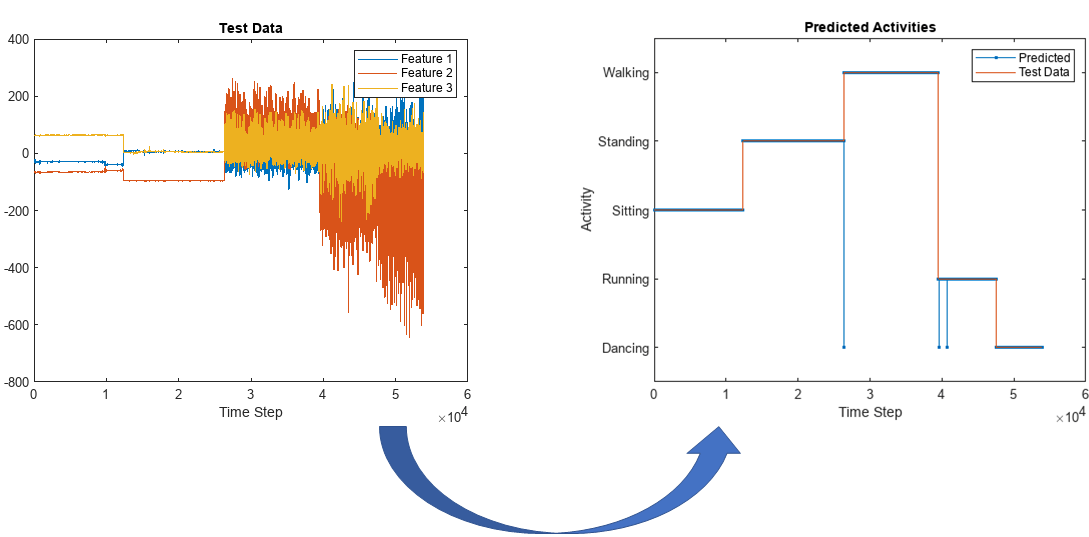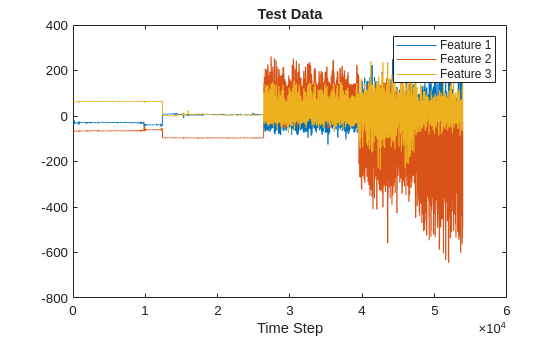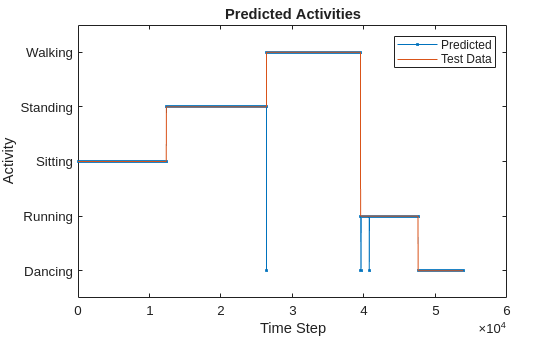# Run Sequence-to-Sequence Classification on Intel FPGA

This example shows how to create, compile, and deploy a long short-term memory (LSTM) network trained on accelerometer data from human movement by using the Deep Learning HDL Toolbox™ Support Package for Intel® FPGA and SoC. Use the deployed network to classify human activity based on sequence input data. Use MATLAB® to retrieve the prediction results from the target device.

This example uses the network trained in the Sequence-to-Sequence Classification Using Deep Learning. This example uses sensor data obtained from a smartphone worn on the body and deploys an LSTM network trained to recognize the activity of the wearer based on time series data that represents accelerometer readings in three different directions. The graphs below show the raw data for these accelerometer readings over time and the resulting classifications. The training data contains time series data for seven people. Each sequence has three features and varies in length. The data set contains six training observations and one test observation.### Prerequisites

• Intel Arria® 10 SoC development board

• Deep Learning HDL Toolbox™ Support Package for Intel® FPGA and SoC

• Deep Learning Toolbox™

• Deep Learning HDL Toolbox™

To load the pretrained human body movement network, enter:

`load SequenceToSequenceClassification`

View the layers of the network by using the Deep Network Designer app.

`deepNetworkDesigner(net)`

### Define FPGA Board Interface

Define the target FPGA board programming interface by creating a `dlhdl.Target` object. Specify that the interface is for a Intel board with a JTAG interface.

To create the target object, enter:

`hTarget = dlhdl.Target("Intel");`

To use the JTAG interface, install Intel™ Quartus™ Prime Standard Edition 22.4. To set the Xilinx Vivado tool path, enter:

```hdlsetuptoolpath('ToolName', 'Altera Quartus II', 'ToolPath', 'C:\altera\22.4\quartus\bin64'); ```

### Prepare Network for Deployment

Prepare the network for deployment by creating a `dlhdl.Workflow` object. Specify the network and bitstream name. Ensure that the bitstream name matches the data type and FPGA board. In this example, the target FPGA board is the Intel Arria 10 SOC board. The bitstream uses a `single` data type.

`hW = dlhdl.Workflow('network',net,'Bitstream','arria10soc_lstm_single','Target',hTarget);`

### Compile Network

Run the `compile` method of the `dlhdl.Workflow` object to compile the network and generate the instructions, weights, and biases for deployment. Because the total number of frames exceeds the default value, set the `InputFrameNumberLimit` name-value argument to `10000` to run predictions in chunks of 10,000 frames and prevent timeouts.

`dn = compile(hW,'InputFrameNumberLimit',10000)`
```### Compiling network for Deep Learning FPGA prototyping ... ### Targeting FPGA bitstream arria10soc_lstm_single. ### The network includes the following layers: 1 'sequenceinput' Sequence Input Sequence input with 3 dimensions (SW Layer) 2 'lstm' LSTM LSTM with 200 hidden units (HW Layer) 3 'fc' Fully Connected 5 fully connected layer (HW Layer) 4 'softmax' Softmax softmax (SW Layer) 5 'classoutput' Classification Output crossentropyex with 'Dancing' and 4 other classes (SW Layer) ### Notice: The layer 'sequenceinput' with type 'nnet.cnn.layer.ImageInputLayer' is implemented in software. ### Notice: The layer 'softmax' with type 'nnet.cnn.layer.SoftmaxLayer' is implemented in software. ### Notice: The layer 'classoutput' with type 'nnet.cnn.layer.ClassificationOutputLayer' is implemented in software. ### Compiling layer group: lstm.wi ... ### Compiling layer group: lstm.wi ... complete. ### Compiling layer group: lstm.wo ... ### Compiling layer group: lstm.wo ... complete. ### Compiling layer group: lstm.wg ... ### Compiling layer group: lstm.wg ... complete. ### Compiling layer group: lstm.wf ... ### Compiling layer group: lstm.wf ... complete. ### Compiling layer group: fc ... ### Compiling layer group: fc ... complete. ### Allocating external memory buffers: offset_name offset_address allocated_space _______________________ ______________ ________________ "InputDataOffset" "0x00000000" "4.0 MB" "OutputResultOffset" "0x00400000" "4.0 MB" "SchedulerDataOffset" "0x00800000" "4.0 MB" "SystemBufferOffset" "0x00c00000" "20.0 MB" "InstructionDataOffset" "0x02000000" "4.0 MB" "FCWeightDataOffset" "0x02400000" "4.0 MB" "EndOffset" "0x02800000" "Total: 40.0 MB" ### Network compilation complete. ```
```dn = struct with fields: weights: [1×1 struct] instructions: [1×1 struct] registers: [1×1 struct] syncInstructions: [1×1 struct] constantData: {} ddrInfo: [1×1 struct] ```

To deploy the network on the Intel Arria 10 SoC hardware, run the `deploy` method of the `dlhdl.Workflow` object. This function uses the output of the `compile` function to program the FPGA board and download the network weights and biases. The `deploy` function programs the FPGA device and displays progress messages, and the required time to deploy the network.

` deploy(hW)`
```### FPGA bitstream programming has been skipped as the same bitstream is already loaded on the target FPGA. ### Deep learning network programming has been skipped as the same network is already loaded on the target FPGA. ```

### Load Human Activity Test Data

Load the test data and classify the activity at each time step. Each sequence has three features and varies in length. The three features correspond to the accelerometer readings in three different directions.

Load the human activity test data. `XTest` contains a single sequence of dimension 3. `YTest` contains a sequence of categorical labels that correspond to the activity at each time step.

```load HumanActivityTest numFeatures = 3; figure plot(XTest{1}') xlabel("Time Step") legend("Feature " + (1:numFeatures)) title("Test Data")```### Run the Prediction

Classify the test data by using the `classify` function.

`YPred = classify(hW.Network, XTest{1});`

Calculate the accuracy of the prediction.

`acc = sum(YPred == YTest{1})./numel(YTest{1})`
```acc = 0.9995 ```

Compare the predictions with the test data by using a plot.

```figure plot(YPred,'.-') hold on plot(YTest{1}) hold off xlabel("Time Step") ylabel("Activity") title("Predicted Activities") legend(["Predicted" "Test Data"])```Compare this graph to the output of the `predict` method.

Run the `predict` method of the `dlhdl.Workflow` object, to retrieve the hardware prediction results.

`predictions = hW.predict(XTest{1}(:,1:10000),Profile='on');`
```### Resetting network state. ### Finished writing input activations. ### Running a sequence of length 10000. Deep Learning Processor Profiler Performance Results LastFrameLatency(cycles) LastFrameLatency(seconds) FramesNum Total Latency Frames/s ------------- ------------- --------- --------- --------- Network 18463 0.00012 10000 185724803 8076.5 memSeparator_0 78 0.00000 lstm.wi 3838 0.00003 lstm.wo 3909 0.00003 lstm.wg 3828 0.00003 lstm.wf 3938 0.00003 lstm.sigmoid_1 295 0.00000 lstm.sigmoid_3 267 0.00000 lstm.tanh_1 267 0.00000 lstm.sigmoid_2 267 0.00000 lstm.multiplication_2 297 0.00000 lstm.multiplication_1 267 0.00000 lstm.c_add 251 0.00000 lstm.tanh_2 271 0.00000 lstm.multiplication_3 261 0.00000 fc 429 0.00000 * The clock frequency of the DL processor is: 150MHz ```
`predictions = horzcat(predictions, hW.predict(XTest{1}(:,10001:20000),Profile='on'));`
```### Resetting network state. ### Finished writing input activations. ### Running a sequence of length 10000. Deep Learning Processor Profiler Performance Results LastFrameLatency(cycles) LastFrameLatency(seconds) FramesNum Total Latency Frames/s ------------- ------------- --------- --------- --------- Network 18363 0.00012 10000 185714233 8076.9 memSeparator_0 78 0.00000 lstm.wi 3828 0.00003 lstm.wo 3799 0.00003 lstm.wg 3818 0.00003 lstm.wf 3929 0.00003 lstm.sigmoid_1 264 0.00000 lstm.sigmoid_3 317 0.00000 lstm.tanh_1 267 0.00000 lstm.sigmoid_2 267 0.00000 lstm.multiplication_2 267 0.00000 lstm.multiplication_1 307 0.00000 lstm.c_add 251 0.00000 lstm.tanh_2 271 0.00000 lstm.multiplication_3 251 0.00000 fc 449 0.00000 * The clock frequency of the DL processor is: 150MHz ```
`predictions = horzcat(predictions, hW.predict(XTest{1}(:,20001:30000),Profile='on'));`
```### Resetting network state. ### Finished writing input activations. ### Running a sequence of length 10000. Deep Learning Processor Profiler Performance Results LastFrameLatency(cycles) LastFrameLatency(seconds) FramesNum Total Latency Frames/s ------------- ------------- --------- --------- --------- Network 18512 0.00012 10000 185720452 8076.7 memSeparator_0 78 0.00000 lstm.wi 3898 0.00003 lstm.wo 3859 0.00003 lstm.wg 3868 0.00003 lstm.wf 4018 0.00003 lstm.sigmoid_1 284 0.00000 lstm.sigmoid_3 267 0.00000 lstm.tanh_1 267 0.00000 lstm.sigmoid_2 257 0.00000 lstm.multiplication_2 257 0.00000 lstm.multiplication_1 257 0.00000 lstm.c_add 251 0.00000 lstm.tanh_2 271 0.00000 lstm.multiplication_3 251 0.00000 fc 429 0.00000 * The clock frequency of the DL processor is: 150MHz ```
`predictions = horzcat(predictions, hW.predict(XTest{1}(:,30001:40000),Profile='on'));`
```### Resetting network state. ### Finished writing input activations. ### Running a sequence of length 10000. Deep Learning Processor Profiler Performance Results LastFrameLatency(cycles) LastFrameLatency(seconds) FramesNum Total Latency Frames/s ------------- ------------- --------- --------- --------- Network 18472 0.00012 10000 185722743 8076.6 memSeparator_0 78 0.00000 lstm.wi 3858 0.00003 lstm.wo 3888 0.00003 lstm.wg 3838 0.00003 lstm.wf 4009 0.00003 lstm.sigmoid_1 264 0.00000 lstm.sigmoid_3 267 0.00000 lstm.tanh_1 270 0.00000 lstm.sigmoid_2 264 0.00000 lstm.multiplication_2 257 0.00000 lstm.multiplication_1 267 0.00000 lstm.c_add 251 0.00000 lstm.tanh_2 271 0.00000 lstm.multiplication_3 261 0.00000 fc 429 0.00000 * The clock frequency of the DL processor is: 150MHz ```
`predictions = horzcat(predictions, hW.predict(XTest{1}(:,40001:50000),Profile='on'));`
```### Resetting network state. ### Finished writing input activations. ### Running a sequence of length 10000. Deep Learning Processor Profiler Performance Results LastFrameLatency(cycles) LastFrameLatency(seconds) FramesNum Total Latency Frames/s ------------- ------------- --------- --------- --------- Network 18475 0.00012 10000 185720273 8076.7 memSeparator_0 78 0.00000 lstm.wi 3858 0.00003 lstm.wo 3879 0.00003 lstm.wg 3849 0.00003 lstm.wf 4019 0.00003 lstm.sigmoid_1 285 0.00000 lstm.sigmoid_3 257 0.00000 lstm.tanh_1 267 0.00000 lstm.sigmoid_2 277 0.00000 lstm.multiplication_2 257 0.00000 lstm.multiplication_1 257 0.00000 lstm.c_add 251 0.00000 lstm.tanh_2 261 0.00000 lstm.multiplication_3 251 0.00000 fc 429 0.00000 * The clock frequency of the DL processor is: 150MHz ```
`predictions = horzcat(predictions, hW.predict(XTest{1}(:,50001:end),Profile='on'));`
```### Resetting network state. ### Finished writing input activations. ### Running a sequence of length 3888. Deep Learning Processor Profiler Performance Results LastFrameLatency(cycles) LastFrameLatency(seconds) FramesNum Total Latency Frames/s ------------- ------------- --------- --------- --------- Network 18253 0.00012 3888 72208234 8076.6 memSeparator_0 78 0.00000 lstm.wi 3838 0.00003 lstm.wo 3798 0.00003 lstm.wg 3838 0.00003 lstm.wf 3899 0.00003 lstm.sigmoid_1 265 0.00000 lstm.sigmoid_3 267 0.00000 lstm.tanh_1 267 0.00000 lstm.sigmoid_2 287 0.00000 lstm.multiplication_2 257 0.00000 lstm.multiplication_1 257 0.00000 lstm.c_add 251 0.00000 lstm.tanh_2 271 0.00000 lstm.multiplication_3 251 0.00000 fc 429 0.00000 * The clock frequency of the DL processor is: 150MHz ```
```save("hardwarepredictions.mat","predictions") indices = []; actions = []; for x = 1:length(YPred) [r,i] = max(predictions(:,x)); indices = [indices i]; switch i case 1 actions = [actions categorical("Dancing")]; case 2 actions = [actions categorical("Running")]; case 5 actions = [actions categorical("Walking")]; case 4 actions = [actions categorical("Standing")]; case 3 actions = [actions categorical("Sitting")]; end end```

Calculate the accuracy of the FPGA board prediction.

`accFPGA = sum(actions == YTest{1})./numel(YTest{1})`
```accFPGA = 0.9958 ```

Plot the comparison between the FPGA board predictions and test data.

```figure plot(actions,'.-') hold on plot(YTest{1}) hold off xlabel("Time Step") ylabel("Activity") title("Predicted Activities") legend(["Predicted" "Test Data"])```The hardware-predicted activities are similar to the activities classified by the `classify` function.